Refer to our Texas Go Math Grade 8 Answer Key Pdf to score good marks in the exams. Test yourself by practicing the problems from Texas Go Math Grade 8 Unit 7 Study Guide Review Answer Key.

Question 1.
Sheri is going to take out a loan for $4,000 that she plans to pay back in 2 years. She wants to know how much more it will cost her in interest if she uses her credit card at 18% interest instead of borrowing from the bank at 10% interest. Both loans require monthly payments. Use an online calculator to find the total repayment for each loan and the difference in the cost of these two choices. (Lesson 16.1) Answer: Given that, Sheri took a loan =$4,000.
Sheri plans to come back in = 2 years.
Interest if she uses her credit card is = 18%.
Formula for simple interest equal to SI = PTR/100
Here,
P = actual money = $4000 T = time = 2 years. R = interest rate = 18% Simple interest for using her credit card =$4000 × 2 × 18/100 = 1440.
2 years = 24 months.
For 1 month = 1440/24 = 60.
Total replacement of loan for 1 month = $4000 + 60 =$4060
Interest if she borrowing from the bank is 10%
P = actual money = $4000 T = time = 2 years. R = interest rate = 10% Simple interest for her borrowing from the bank is =$4000 × 2 × 10/100 = 800.
2 years = 24 months.
For 1 month = 800/24 = 33.3
Total replacement of loan for 1 month = $4000 + 33.3 =$4033.3
Difference in the cost of two loans for 1 month is $4060 –$4033.3 = $26.7. The difference between the two loans is$26.7.

Question 2.
You are trying to decide which account to put $3,500 into for the next 6 years. One account has an interest rate of 2.9%, compounded annually. The other account has a simple interest rate of 3.1 %. Which account will earn more interest over 6 years, and how much more interest will it earn? (Lesson 16.2) Answer: Given that, The total amount =$3500.
The total number of years = 6 years.
One account an interest rate = 2.9% in compound interest.
Formula for compound interest = A = P(1 + R/1000)n
P = actual money = $3500 n = time = 6 years. R = interest rate = 2.9% Compound interest for 6 years = A = 3500(1 + 3.1/100)6 = 3500(1 + 0.031)6 = 3500(1.031)6 = 3500(1.20) =$4200
The other account = 3.1% in simple interest.
Formula for simple interest equal to SI = PTR/100
Here,
P = actual money = $3500 T = time = 6 years. R = interest rate = 3.1% Simple interest for 6 years =$3500 × 6 × 3.1/100 = $651. The difference between the two loans is$4200 – $651 =$3549
The simple interest loan is $3549 more interest than compound interest. Question 3. Maria has$ 120 to spend on food for the week. She goes out to a restaurant to eat dinner with her friends and spends $62 on the meal. Did Maria make a financially responsible decision or a financially irresponsible decision? Explain your answer. (Lesson 16.3) Answer: Given that, Maria spends on food for the week =$120.
1 week = 7 days
For 1 day meal = $120/7 =$17.14.
Maria goes out to a restaurant to eat dinner with friends and spends on meal = $62. It is an irresponsible decision for her to go out to a restaurant and to spend$62 on a meal.
Maria spending $120 on food for the week is a responsible decision. Question 4. Use an online tool to estimate the cost for one year at a 4-year university and one year at a 2-year college in Texas. (Lesson 16.4)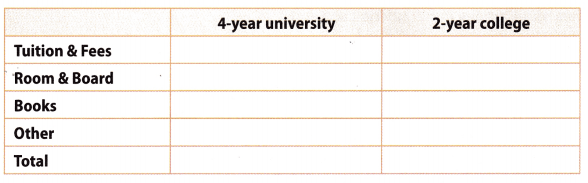a. Find the cost of attending the university for four years. Answer: Tuition and fees for 4 years university = 24,000. Room and board for 4 year university = 14,000 Books for 4 year university = 1000 Others for 4 year university = 500 Tuition and fees for 2 years college = 12,000. Room and board for 2 year college = 7,000 Books for 2 year college = 6000 Others for 2 year college = 300 The total cost of attending the university for 4 years = 24,000 + 14,000 + 1000 + 500 = 39,500. b. Find the cost of attending the two-year college and transferring to the university for your final two years of school. Answer: Tuition and fees for 4 years university = 24,000. For 2 years = 12000 Room and board for 4 year university = 14,000 For 2 years = 7000 Books for 4 year university = 1000 For 2 years = 500. Others for 4 year university = 500 For 2 years = 250. Tuition and fees for 2 years college = 12,000. Room and board for 2 year college = 7,000 Books for 2 year college = 6000 Others for 2 year college = 300 The cost of attending the two-year college and transferring to the university for your final two years of school = 1200 + 7000 + 500 + 250 + 12000 + 7000 + 6000 + 300 = 45,050 Texas Go Math Grade 8 Unit 7 Performance Tasks Answer Key Question 1. CAREERS IN MATH Organic Farmer Carlos is an organic farmer, and his business is doing so well that he his thinking of expanding in the next few years. He decides to start saving for this expansion and is going to put$8,200 into a savings account. At his credit union, he has two choices for savings accounts: Simple Savers that earns 2% simple interest per year, and Super Savers which earns 1.95% interest, compounded annually.
a. How much will Carlos have after 2 years if he chooses the Simple Savers account? Show your work.
Given that,
Organic Farmer Carlos’ money in the savings account is $8200. Simple Savers that earns 2% simple interest per year. Formula for simple interest equal to SI = PTR/100 Here, P = actual money =$8200
T = time = 2 years.
R = interest rate = 2%
Simple interest for 2 years = $8200 × 2 × 2/100 =$328.

b. How much will Carlos have after 2 years if he chooses the Super Savers account? Show your work.
Given that,
Organic Farmer Carlos’ money in the savings account is $8200. Super savers that earn 1.95 compounded interest per year. Formula for compound interest = A = P(1 + R/100)n P = actual money =$8200
n = time = 2 years.
R = interest rate = 1.95%
Compound interest for 2 years = A = 8200(1 + 1.95/100)2
= 8200(1 + 0.0195)2
= 8200(1.0195)2
= 8200(1.039)
Compounded interest for 2 years = $8519.8 c. Which account would you recommend Carlos use and why? Answer: Simple interest for 2 years =$8200 × 2 × 2/100 = $328. Compounded interest for 2 years =$8519.8
I recommend the simple interest because its interest is less than the compounded interest.

d. If Carlos decides to keep the money in the savings account for 5 years, would you change your recommendation? Why or why not?
Organic Farmer Carlos’ money in the savings account is $8200. Simple Savers that earn 2% simple interest per year. Formula for simple interest equal to SI = PTR/100 Here, P = actual money =$8200
T = time = 5 years.
R = interest rate = 2%
Simple interest for 2 years = $8200 × 2 × 5/100 =$820.
Organic Farmer Carlos’ money in the savings account is $8200. Super savers that earn 1.95 compounded interest per year. Formula for compound interest = A = P(1 + R/100)n P = actual money =$8200
n = time = 2 years.
R = interest rate = 1.95%
Compound interest for 2 years = A = 8200(1 + 1.95/100)5
= 8200(1 + 0.0195)5
= 8200(1.0195)5
= 8200(1.101)
Compounded interest for 2 years = $9028.2. There is no change in the recommendation because after 5 years also simple interest is less than compounded interest. Question 2. Kay wants a new television. She sees an advertisement in the newspaper for a rent-to-own store, where for$80 a month she can rent a new television. And, if she rents for 18 months, she will own the television outright. Kay is considering this option, because she doesn’t have enough money to purchase a television but she can pay $80 a month. a. If Kay rents the television for 18 months, how much will she pay in total for the television? Answer: Given that, Kay can rent a new television for 1 month =$80.
For 18 months = $80 × 18 =$1440.
Kay rents for 18 months = $1440. b. The same television sells for$429 at an electronics store. How much more will Kay end up paying if she rents the television for 18 months than if she buys it outright?
Given that,
The television sells at an electronic store is $429 Kay can rent a new television for 1 month =$80.
For 18 months = $80 × 18 =$1440.
Kay rents for 18 months = $1440. Subtract$1440 – $429 =$1011.
Key will end up paying if she rents for 18 months = $1011. c. What financially responsible recommendation would you give to Kay about purchasing the television? Answer: I recommend that you purchase the television at the electronics shop. Because the cost of the television in the electronic shop is less than the rent for 18 months. Question 3. Anastasia is a high school senior who wants to be an architect. She was accepted at a four-year university and was offered a scholarship of$17,800 per year. The costs per year at this university are shown in the table.She can also attend a community college for the first two years. The tuition for the community college is $1,150 per year. She would need to rent an apartment and buy her food, which she estimates will cost$400 a month for the apartment and $210 a month for food. She would still need to buy books and materials at$850 a year.

a. How much will it cost Anastasia to attend the university for all four years of college, assuming she has her scholarship all four years, and she does not pay for housing and food during the summers? Show how you got your answer.
Given that,
The amount of Anastasia scholarship is = $17800 per year. For four years = 4 ×$17800 = $71200 The total amount of tuition fee for one year is$17400
The total amount of tuition fee for four years = $17400 × 4 =$69600
The total amount of fee for room and board = $10,350 For four years = 4 ×$10,350 = $41400 The total amount of fee for books and materials =$850
For four years = $850 × 4 =$3400
The total amount for the community tuition = $1150 per year. For two years =$1150 × 2 = $2300 The total amount for the apartment =$400 per month
There is no need to pay money in the summer for food and an apartment.
1 year = 12 months in that 3 months are summer so 12 – 3 – 9.
For 4 years = 4 × 9 = 36 months.
For 4 years = 36 × $400 =$14400
The total amount for the food = $210 per month For 4 years = 36 months. For 4 years = 36 ×$210 = $7560 She still needs to buy the books and materials =$850.
For 4 years = 4 × $850 =$3400
The total cost Anastasia to attend the university for all four years of college = $71200 +$69600 + $41400 +$3400 + $2300 +$14400 + $7560 +$3400 = $213,260 b. How much will it cost Anastasia to attend the community college for the first two years, and to attend the university for the last two years? Show your work. Answer: Given that, The total amount of tuition fee for one year is$17400
The total amount of tuition fee for the last two years = $17400 × 2 =$34800
The total amount for the community tuition = $1150 per year. For two years =$1150 × 2 = $2300. The total cost for Anastasia to attend the community college for the first two years, and to attend the university for the last two years =$34800 + $2300 =$37100.

c. Give one reason in favor of going to the university for her entire college career, and one reason in favor of going two years to the community college and two years to the university.
Given that,
The total amount of tuition fee for one year is $17400 The total amount of tuition fee for four years =$17400 × 4 = $69600 The total cost for Anastasia to attend the community college for the first two years, and to attend the university for the last two years =$34800 + $2300 =$37100.
The fee for four years of university college is more than the community college of two years and two years of the university college.

Texas Go Math Grade 8 Unit 7 Mixed Review Texas Test Prep Answer Key

Selected Response

Question 1.
Which interest rate and time period result in the lowest total loan repayment for a $5,000 loan earning simple interest? (A) 3 years at 10% (B) 3 years at 13% (C) 4 years at 8% (D) 4 years at 11% Answer: (A) 3 years at 10% Formula for simple interest equal to SI = PTR/100 Here, P = actual money =$5000
T = time = 3 years.
R = interest rate = 10%
Simple interest for 3 years = $5000 × 3 × 10/100 =$1500.

(B) 3 years at 13%
Formula for simple interest equal to SI = PTR/100
Here,
P = actual money = $5000 T = time = 3 years. R = interest rate = 13% Simple interest for 3 years =$5000 × 3 × 13/100 = $1950 (C) 4 years at 8% Formula for simple interest equal to SI = PTR/100 Here, P = actual money =$5000
T = time = 4 years.
R = interest rate = 8%
Simple interest for 4 years = $5000 × 4 × 8/100 =$1600
(D) 4 years at 11%
Formula for simple interest equal to SI = PTR/100
Here,
P = actual money = $5000 T = time = 4 years. R = interest rate = 11% Simple interest for 4 years =$5000 × 4 × 11/100 = $2200 Option A is the correct answer. Question 2. Which of the relations below is a function?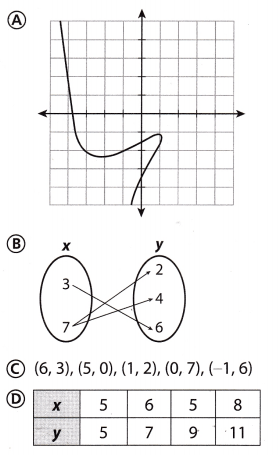Answer: Option C is a function Question 3. Amanda used her PIN to complete a transaction at a department store. Which payment method does this describe? (A) gift card (B) credit card (C) debit card (D) personal check Answer: A transaction at a department store payment method is a credit card. A credit card is the card issued by the bank and it is used to purchase goods or services on credit. Option B is the correct answer. Question 4. What is the surface area of the rectangular prism?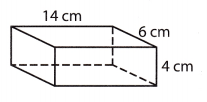(A) 160 cm2 (B) 164 cm2 (C) 328 cm2 (D) 392 cm2 Answer: Formula for the surface area of the rectangular prism = 2(lw + lh + wh) Length = 14cm Width = 6cm Height = 4cm Surface area of the rectangular prism = 2(14 × 6 + 14 × 4 + 6 × 4) = 2(84 + 56 + 24) = 2(164) = 328. The surface area of the rectangular prism = 328 cm2 Option C is the correct answer. Question 5. Rianna opens a savings account with$900. Her account earns interest at a rate of 1.3%, compounded annually. How much money is in the account after 4 years?
(A) $46.80 (B)$47.72
(C) $946.80 (D)$947.72
Given that,
Rianna opens a savings account = $900. Interest at rate = 1.3%. The total number of years = 4. Formula for compound interest = A = P(1 + R/100)n P = actual money =$900
n = time = 4 years.
R = interest rate = 1.3%
Compound interest for 4 years = A = 900(1 + 1.3/100)4
= 900(1 + 0.013)4
= 900(1.013)4
= 900(1.053022)
= $947.72 Money in the account after 4 years =$947.72.
Option D is the correct answer.

Hot Tips! Some answer choices, called distracters, may seem correct because they are based on common errors made in calculations.

Question 6.
Richard took a handful of pencils from a large box. Out of the 15 pencils in his hand, 4 were glittery. How many glittery pencils should Richard expect to find in the box if there are a total of 240 pencils?
(A) 16 glittery pencils
(B) 32 glittery pencils
(C) 64 glittery pencils
(D) 96 glittery pencils
Given that,
Richard took a handful of pencils from a large box.
Out of 15 pencils, 4 are glittery.
Richard expects to find 240 pencils = 240/15 = 16.
There are 16 glittery pencils in the 240 pencils.
Option A is the correct answer.

Question 7.
Yvonne started running 8 minutes after Cassie started. Cassie was running at a rate of 500 feet per minute. Yvonne was running at a rate of 600 feet per minute. Which equation could you solve to find how long it will take Yvonne to catch up to Cassie?
(A) 600t + 3 = 500t
(B) 600t + 4,800 = 500t
(C) 500t + 3 = 600t
(D) 500t + 4,000 = 600t
Given that,
Cassie was running at a rate of 500 feet per minute.
Yvonne started running after 8 minutes of Cassie.
Yvonne was running at a rate of 600 feet per minute.
1 minute = 600 feets.
8 minutes = 6 × 600 = 4800 feets.
Yvonne to catch up to cassie = 4800 feet.
Option B is the correct answer.

Question 8.
Leah is planning on attending a public university to earn a four year bachelor’s degree. For one year, the tuition and fees are $10,220, room and board is$6250, and books are $540. At these rates, how much should Leah expect four years of school to cost? (A)$16,470
(B) $17,010 (C)$34,020
(D) $68,040 Answer: Given that, Leah tuition and fees for one year =$10220.
For room and board = $6250. For books for one year =$540.
The total amount for one year = $10220 +$6250 + $540 =$17010
Leah expects four years of school = $17010 × 4 =$68040.
Option D is the correct answer.

Question 9.
The square below is dilated under the dilation (x, y) →(0.25x, 0.25y).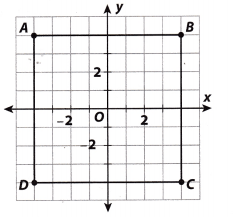What are the coordinates of A’?
(A) (-4, -4)
(B) (-1, -1)
(C) (-2, -2)
(D) (4, -4)
Dilation means changing the positions and size of a figure but not the shape.
Coordinates of A(-4,4), B(4,4), C(4,-4) D(-4,-4)
The coordinates of A’ is (4,-4).

Gridded Response

Question 10.
Fletcher puts $4,500 in a savings account earning 1.5% interest compounded annually. He does not make any deposits or withdrawals for 3 years. How much interest does the account earn? Round to the nearest cent.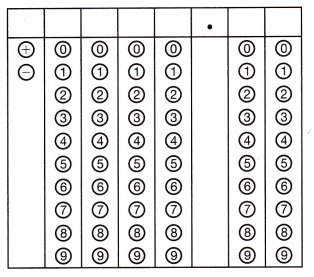Answer: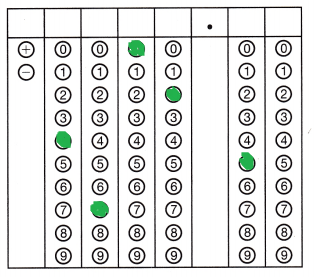Given that, Fletcher puts in a savings account =$4500.
Interest compounded annually = 1.5%.
The total number of years = 3
Formula for compound interest = A = P(1 + R/100)n
P = actual money = $4500 n = time = 3 years. R = interest rate = 1.5% Compound interest for 3 years = A = 4500(1 + 1.5/100)³ = 4500(1 + 0.015)³ = 4500(1.015)³ = 4500(1.045) = 4702.5 Hot Tips! Underline key words given in the test question so you know for certain what the question is asking. Question 11. Pat puts$1,310 in a savings account earning 2% simple interest and does not make any deposits or withdrawals for 8 years. How much interest does the account earn?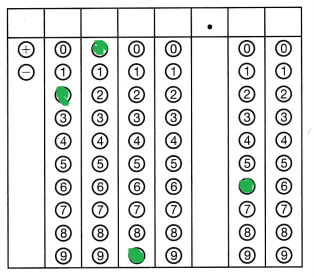Given that,
Pat puts in a savings account = $1310. Interest = 2% Total number of years = 8 years. Formula for simple interest equal to SI = PTR/100 Here, P = actual money =$1310
T = time = 8 years.
R = interest rate = 2%
Simple interest for 8 years = 1310 × 8 × 2/100 = $209.6 Interest for the amount =$209.6

Use the puzzle to preview key vocabulary from this unit. Unscramble the circled letters within found words to answer the riddle at the bottom of the page.Across
2. College funding awards for students based on achievement. (Lesson 1.4)
4. Payment card you can use to make purchases, and the money is deducted immediately from a bank account. (Lesson 16.4)
5. College funding awards from the government or other organizations, usually for students who need money the most. (Lesson 16.4)

Down
1. The original amount of money deposited or saved. (Lesson 16.2)
3. Payment card you can use to make purchases, then pay a bill at the end of a billing cycle. (Lesson 16.4)

Question.
Why did the man put his money in the freezer?